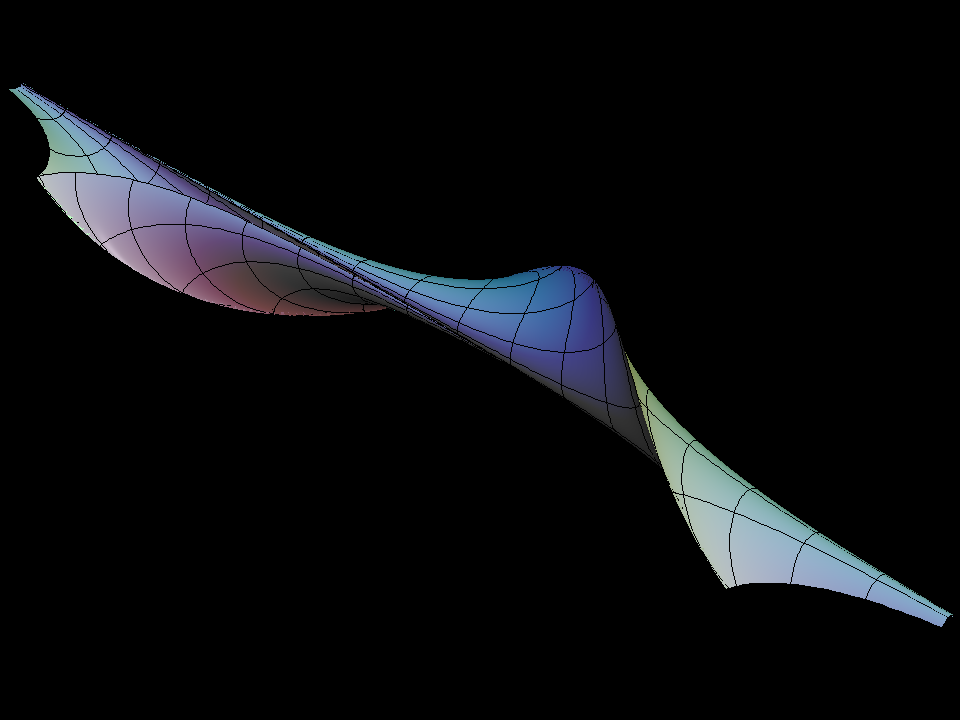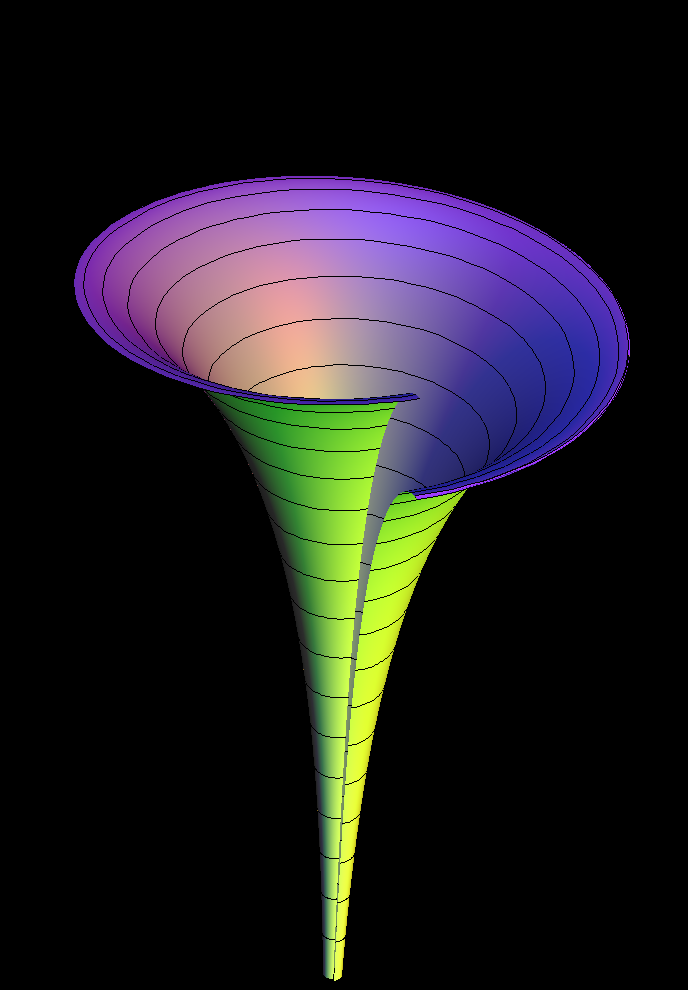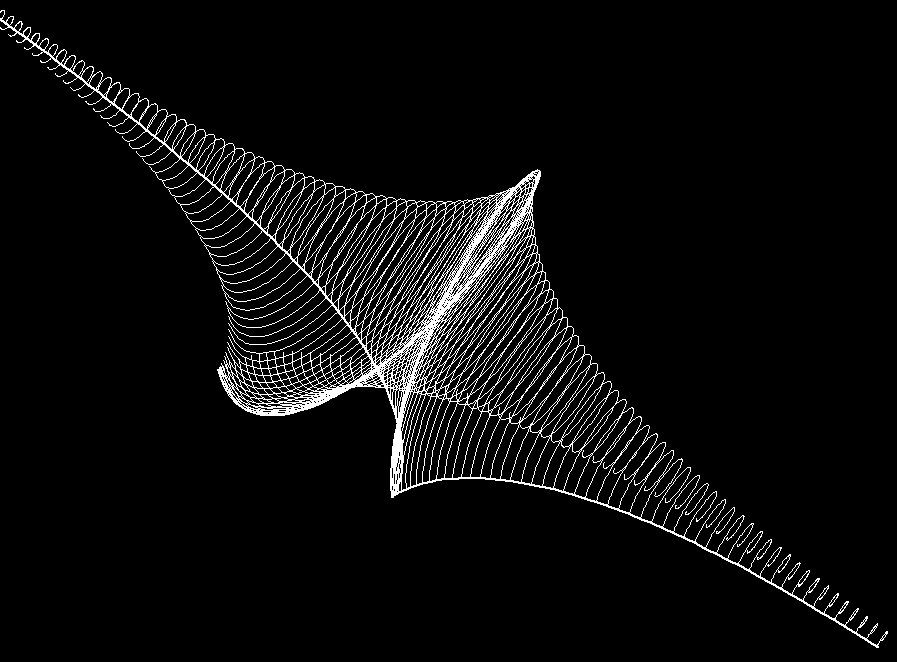# Dini Surface

Dini surface is a family of surfaces that have constant Gaussian curvature of -1. We write `K = -1`.

(K = -1 surfaces are also called pseudospherical surfaces in general, because the unit sphere has constant Gaussian curvature of 1.)

Many explicitly parametrized surfaces of `K = -1` were found in the 1800s, among them the Dini family.

Today we have a general theory which associates with every soliton solution of the sine-Gordon equation `q_xt = sin(q(x,t))` a `K = -1` surface. In this context the Dini family comes from the 1-soliton solution `q(x,t) = 4arctan(exp(ax + t/a)`. These solutions are also called Kink solutions.“Pseudosphere splits into Dini surface family” This animation shows how the pseudosphere splits open along a longitude line and deforms into the Dini family. Only a small part of the Dini surfaces is drawn because the full surface develop self-intersections that makes it hard to understand. These self-intersections do not occur if we show just half a pseudosphere.

[see Pseudosphere]“half dini grow” This animation shows a Dini surface close to half a pseudosphere. The multicovered pseudosphere is deformed into a corkscrew like shape. The half Dini surface can be extended without developing self-intersections! The full half dini surface is isometric to a horodisk of the hyperbolic plane.

In hyperbolic space H^2 there are limits of circles with the radius going to infinity and one diameter endpoint is kept fixed. These limit circles are called horocircles and their insides are called horodisks.Curvature lines of Dini surface. The thick line, drawn pole to pole, is a curvature line. The other curvature lines (spiral-like) are orthogonal to it. (Principle curvature lines are always orthogonal by Euler's theorem.)

The soliton solution `q(x,t)` determines first and second fundamental form of the (K = -1) surface. These give ODEs for the curvature lines.

Dini surface is also known as the one-soliton surface.

```     Dini Surface Parametric Equations

x = (u - t)
y = (r * cos(v))
z = (r * sin(v))

Where:

psi := aa;
if (psi < 0.001) then  psi := 0.001;
if (psi > 0.999) then psi := 0.999;
psi := psi * pi;
sinpsi := sin(psi);
cospsi := cos(psi);
g := (u - cospsi * v) / sinpsi;
s := exp(g);
r := (2 * sinpsi) / (s + 1 / s);
t := r * (s - 1 / s) * 0.5;
```